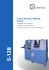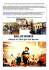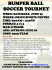# The moment of a force or the torque about an axis

## Transcription

The moment of a force or the torque about an axis
```The moment of a force or the torque about an axis
The force is exerted on the ball,
the ball moves from an initial
position to a final position.
The ball is said to have
undergone a translational
motion.
When the force is exerted on the door, it will rotate or turn. In this case the
force produces a turning effect which is known as a torque or moment of a
force. Thus, the torque of the force about a give axis of rotation is given by
a product of the force and a perpendicular distance from the axis of rotation.
Mathematically,
Units are Nm
=×
The distance is called a lever arm of the turning effect.
∝
∝
Application of torque
A mechanic is loosening a tight
nut. He could ask a colleague to
assist him exerting more force.
That will increase a turning
effect or a torque on a nut.
Instead, he decided to use an
extension pipe to increase the
lever arm (r) and thereby
increasing a torque ().
=×
Principle of moment
Consider a uniform bar resting on a triangular block, as shown in the figure below. Let the rotation axis or the pivot
be at the point of contact between the bar and the triangular block, which is at the centre of the bar. Two objects are
suspended at the ends of the bar and they have the potential of causing a torque.
N
d2
W2
d1
W1
= × = × The contention is that anticlockwise moments are positive and clockwise moments are negative
Principle of moments
When a body is in equilibrium under the action of any number of coplanar forces, the algebraic sum of the moments
of the forces about any point in the plane is zero. Mathematically,
= − + = − × + × = 0
Principle of moment
Example 3.11: Moment about an axis
Two children are playing on a see-saw. The total length of the see-saw is 4 m and it is pivoted exactly in the middle.
One child weighs 100 N and sits at the end of one side of the see-saw. If the other child weighs 160 N, how far from
the other end must she sit so that the see-saw is balanced.
Rotation axis or the pivot
The equilibrium of a rigid body under the action of a system of coplanar forces
The figure shows a rigid bar AB resting on two supports. R1 and R2 are the reaction (normal) forces at the supports,
and W is the weight of the bar. If the bar is uniform the weight acts at the centre of the bar. Let the axis of rotation
(pivot) be at point A. For a rigid body at equilibrium (i.e. no acceleration),
1.
The vector sum of all the external forces acting on the body must be zero:
∑ = , the considering x- and y-components, ∑ = and ∑ = 2. The algebraic sum of the moments of all the forces about any axis perpendicular to the plane of forces must be
zero (Principle of moments):
.∑ = The net force is:
.∑ = + − = 0
The moment or the torque about A:
∑ = !" − !" = 0
.∑ = ( × \$ + × %) − × ' = 0
Example
D8 A uniform rod 1m long and weighing 30 N is supported in a horizontal position on a pivot with weights of 40 N
and 50 N suspended from its ends. Calculate the position of the pivot.
Centre of gravity
For any rigid body, a centre of gravity is defined as a point from which weight of the body may be considered to act.
The weight around the centre of gravity is balanced.
Irregular objects
Regular objects
Stable, unstable and neutral equilibrium
Stable equilibrium: the ball returns to its original position.
Unstable equilibrium: the ball takes up a new position beyond an
original small displacement.
Neutral equilibrium: The ball takes up a new position at the end of
the displacement.
```

### Kearfott Corporation - Astronautics Corporation of America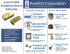### Torque/Cross Product Worksheet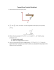### frs_brz_sheet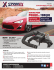### Autogard Series 820 Torque Limiter for Mining Conveyors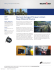### Spinning: Torque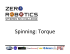### Electric Nutrunner DE1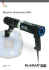### Saturday 22nd September 2012### 5-Axis Turning / Milling Center